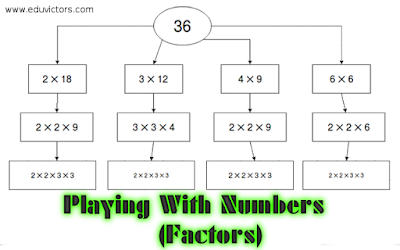## Playing With Numbers NCERT Exercise 3.1

CBSE Class 6 Maths

Q1. Write all the factors of the following numbers :
(a) 24
(b) 15
(c) 21
(d) 27
(e) 12
(f) 20
(g) 18
(h) 23
(i) 36

(a) 24 = 1 × 24
= 2 × 12
= 3 × 8
= 4 × 6
= 6 × 4
∴ Factors of 24 = 1, 2, 3, 4, 6, 12, 24

(b) 15 = 1 × 15
= 3 × 5
= 5 × 3
∴ Factors of 15 = 1, 3, 5, 15

(c) 21 = 1 × 21
= 3 × 7
= 7 × 3
∴ Factors of 21 = 1, 3, 7, 21

(d) 27 = 1 × 27
= 3 × 9
= 9 × 3
∴ Factors of 27 = 1, 3, 9, 27

(e) 12 = 1 × 12
= 2 × 6
= 3 × 4
= 4 × 3
∴ Factors of 12 = 1, 2, 3, 4, 6, 12

(f) 20 = 1 × 20
= 2 × 10
= 4 × 5
= 5 × 4
∴ Factors of 20 = 1, 2, 4, 5, 10, 20

(g) 18 = 1 × 18
= 2 × 9
= 3 × 6
∴ Factors of 18 = 1, 2, 3, 6, 9, 18

(h) 23 = 1 × 23
∴ Factors of 23 = 1, 23

(i) 36 = 1 × 36
= 2 × 18
= 3 × 12
= 4 × 9
= 6 × 6

∴ Factors of 36 = 1, 2, 3, 4, 6, 9, 12, 18, 36

Q2: Write first five multiples of :
(a) 5
(b) 8
(c) 9

(a) 5 × 1 = 5,
5 × 2 = 10,
5 × 3 = 15,
5 × 4 = 20,
5 × 5 = 25
∴ First five multiples of 5 are 5, 10, 15, 20, 25.

(b) 8 × 1 = 8,
8 × 2 = 16,
8 × 3 = 24,
8 × 4 = 32,
8 × 5 = 40
∴ First five multiples of 8 are 8, 16, 24, 32, 40.

(c) 9 × 1 = 9,
9 × 2 = 18,
9 × 3 = 27,
9 × 4 = 36,
9 × 5 = 45
∴ First five multiples of 9 are 9, 18, 27, 36, 45.

Q3: Match the items in column 1 with the items in column 2.
Column 1 Column 2
(i) 35 (a) Multiple of 8
(ii) 15 (b) Multiple of 7
(iii) 16 (c) Multiple of 70
(iv) 20 (d) Factor of 30
(v) 25 (e) Factor of 50
(f) Factor of 20

(i) → (b), (c)
(ii) → (d),
(iii) → (a),
(iv) → (f),
(v) → (e)

Q4: Find all the multiples of 9 upto 100.

Answer: Multiples of 9 up to 100 are: 9, 18, 27, 36, 45, 54, 63, 72, 81, 90, 99